• +91 9971497814
• info@interviewmaterial.com

# RD Chapter 15- Areas of Parallelograms and Triangles Ex-15.3 Interview Questions Answers

### Related Subjects

Question 1 : In the given figure, compute the area of quadrilateral ABCD.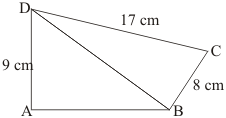Given: Here from the given figure we get
(1) ABCD is a quadrilateral with base AB,
(2) ΔABD is a right angled triangle
(3) ΔBCD is a right angled triangle with base BC right angled at B
To Find: Area of quadrilateral ABCD
Calculation:
In right triangle ΔBCD, by using Pythagoreans theorem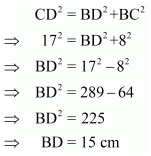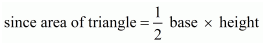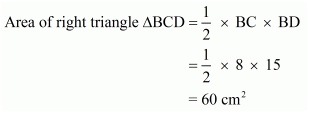In right triangle ABD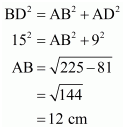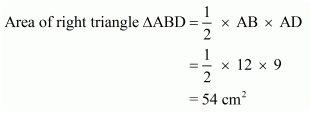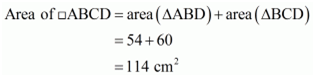Hence we get Area of quadrilateral ABCD =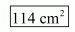Question 2 : In the given figure, PQRS is a square and T and U are, respectively, the mid-points of PS and QR. Find the area of Δ OTS if PQ = 8 cm.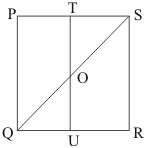Given: Here from the given figure we get
(1) PQRS is a square,
(2) T is the midpoint of PS which means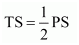(3) U is the midpoint of PS which means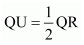(4) QU = 8 cm
To find: Area of ΔOTS
Calculation:
Since it is given that PQ = 8 cm. So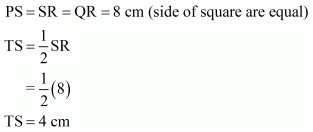Since T and U are the mid points of PS and QR respectively. So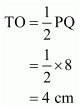Therefore area of triangle OTS is equals to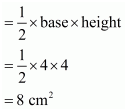Hence we get the result that Area of triangle OTS is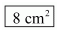Question 3 : Compute the area of trapezium PQRS in the given figure.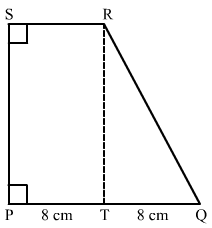Given:
(1) PQRS is a trapezium in which SR||PQ..
(2) PT = 5 cm.
(3) QT = 8 cm.
(4) RQ = 17 cm.
To Calculate: Area of trapezium PQRS.
Calculation:
In triangle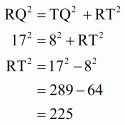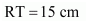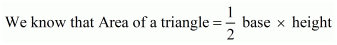.So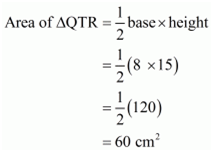No area of rectangle PTRS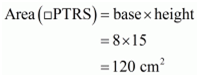Therefore area of trapezium PQRS is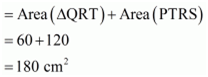Hence the answer is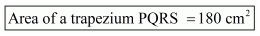Question 4 : In the given figure, ∠AOB = 90°, AC = BC, OA = 12 cm and OC = 6.5 cm. Find the area of Δ AOB.

Given: In figure:
(1) ∠AOB = 90°
(2) AC = BC,
(3) OA = 012 cm,
(4) OC = 6.5 cm.
To find: Area of ΔAOB
Calculation:
It is given that AC = BC where C is the mid point of AB
We know that the mid point of hypotenuse of right triangle is equidistant from the vertices
Therefore
CA = BC = OC
⇒ CA = BC = 6.5
⇒ AB = 2 × 6.5 = 13 cm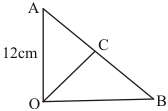Now in triangle OAB use Pythagoras Theorem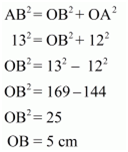So area of triangle OAB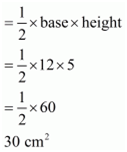Hence area of triangle is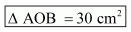Question 5 : In the given figure, ABCD is a trapezium in which AB = 7 cm, AD = BC = 5 cm, DC = x cm, and distance between AB and DC is 4 cm. Find the value of x and area of trapezium ABCD.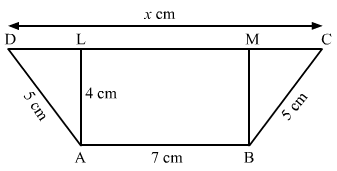Given: Here from the given figure we get
(1) ABCD is a trapezium
(2) AB = 7 cm,
(3) AD = BC = 5 cm,
(4) DC = x cm
(5) Distance between AB and DC is 4 cm
To find:
(a) The value of x
(b) Area of trapezium
Construction: Draw AL⊥ CD, and BM ⊥ CD
Calculation:
Since AL ⊥ CD, and BM ⊥ CD
Since distance between AB and CD is 4 cm. So
AL = BM = 4 cm, and LM = 7 cmIn triangle ADL use Pythagoras Theorem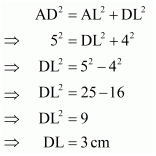Similarly in right triangle BMC use Pythagoras Theorem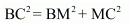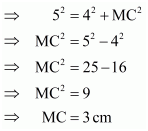Now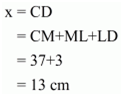We know that,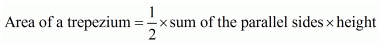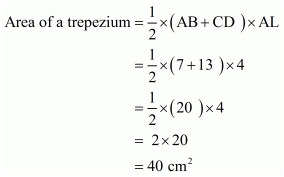We get the result as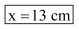Area of trapezium is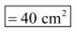Question 6 : In the given figure, OCDE is a rectangle inscribed in a quadrant of a circle of radius 10 cm. If OE = 25–√5, find the area of the rectangle.

Given: Here from the given figure we get
(1) OCDE is a rectangle inscribed in a quadrant of a circle with radius 10cm,
(2) OE = 2√5cm
To find: Area of rectangle OCDE.
Calculation:
In right triangle ΔODE use Pythagoras Theorem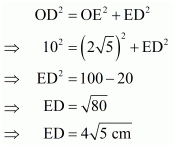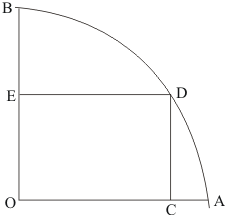We know that,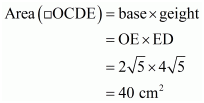Hence we get the result as area of Rectangle OCDE =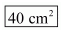Question 7 :
In the given figure, ABCD is a trapezium in which AB || DC. Prove that
ar(Δ AOD) = ar(Δ BOC).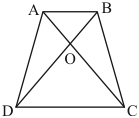Given:
ABCD is a trapezium with AB||DC
To prove: Area of ΔAOD = Area of ΔBOC
Proof:
We know that ‘triangles between the same base and between the same parallels have equal area’Here ΔABC and ΔABD are between the same base and between the same parallels AB and DC.
Therefore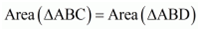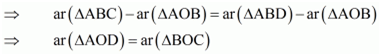Hence it is proved that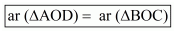Question 8 :
In the given figure, ABCD, ABFE and CDEF are parallelograms. Prove that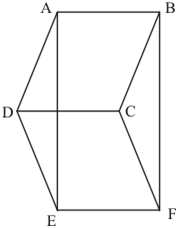Given:
(1) ABCD is a parallelogram,
(2) ABFE is a parallelogram
(3) CDEF is a parallelogram
To prove: Area of ΔADE = Area of ΔBCF
Proof:
We know that,” opposite sides of a parallelogram are equal”
Therefore for
Parallelogram ABFE, AE = BF
Parallelogram CDEF, DE = CF.Thus, in ΔADE and ΔBCF, we have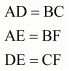So be SSS criterion we have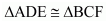This means that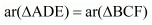Hence it is proved that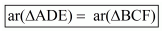Question 9 :
Diagonals AC and BD of a quadrilateral ABCD intersect each other at P. Show that:
ar(Δ APB) ✕ ar (Δ CPD) = ar (Δ APD) ✕ ar (Δ BPC)

Given:
(2) Diagonals AC and BD of quadrilateral ABCD intersect at P.
To prove: Area ofΔ APB ×Area of ΔCPD = Area of ΔAPD × Area of ΔBPC
Construction: Draw AL perpendicular to BD and CM perpendicular to BD
Proof:
We know that
Area of triangle =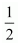× base× height
Area of ÄAPD =. DP . AL …… (1)
Area of ÄBPC =. CM . BP …… (2)
Area of ÄAPB =. BP . AL …… (3)
Area of ÄCPD =. CM . DP …… (4)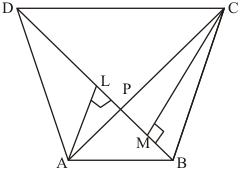Therefore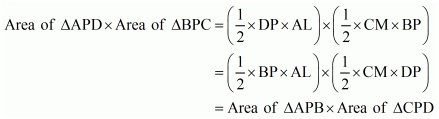Hence it is proved that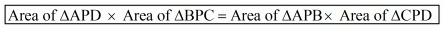Question 10 : In the given figure, ABC and ABD are two triangles on the base AB. If line segment CD is bisected by AB at O, show that ar (Δ ABC) = ar (Δ ABD)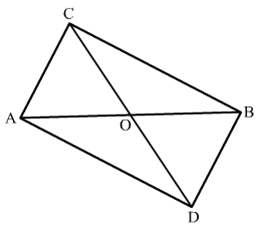Given:
(1) ABC and ABD are two triangles on the same base AB,
(2) CD bisect AB at O which means AO = OB
To Prove: Area of ΔABC = Area of ΔABD
Proof:
Here it is given that CD bisected by AB at O which means O is the midpoint of CD.
Therefore AO is the median of triangle ACD.Since the median divides a triangle in two triangles ofequal area

Therefore Area of ÄCAO = Area of ÄAOD ......(1)

Similarly for Δ CBD, O is the midpoint of CD

Therefore BO is the median of triangle BCD.

Therefore Area of ÄCOB = Area of ÄBOD ......(2)

Adding equation (1) and (2) we get

Area of ΔCAO + Area of ΔCOB = Area of ΔAOD + Area of ΔBOD

Areaof ÄABC =Area of ÄABD

Hence it is proved that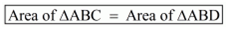Todays Deals### RD Chapter 15- Areas of Parallelograms and Triangles Ex-15.3 Contributorskrishan

Name:
Email:

# Latest News# 9000 interview questions in different categories